# How do you find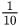of a number?Huda

Solution:

Step:1 The fractioncan be written in the decimal form as 0.1.

Step:2 Therefore, the valueof a number n can be obtained as: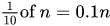Step:3 For example, if number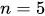then the value corresponding toof this number is calculated as follows: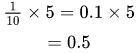Thus, the valueof a number is equal to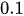of that number.

Answer:The valueof a number is equal toof that number.

Similar Problems:

Problem 1:What is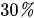of 40?

Solution:

Step:1 The percentage value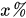is the value x as a fraction of 100, which is written as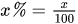.

Step:2 The valueof 40 is therefore equal to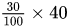.

Step:3 Simplify the above value as shown below:The result is 12.

Answer:Theof 40 is 12.

Problem 2:What is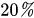of 40?

Solution:
Step:1 The percentage valueis the value x as a fraction of 100, which is written as.

Step:2 The valueof 40 is therefore equal to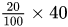.

Step:3 Simplify the above value as shown below:The result is 8.

Answer:Theof 40 is 8.

### Need homework help now?

tutors online

Related Questions
##### (t) in °C of the pot of soup at time t (in The initial temperature of a pot of soup is 80°C. The temperature minutes) satisfies the differential equation dT di T-20 10 (a) Solve the differential equation, and find an explicit expression for the function (). By separating variables, integrating and manipulating arbitrary constants, . We are also told that so that and so . ...
Notes
Me

OR

Don't have an account?

Join OneClass

Access over 10 million pages of study
documents for 1.3 million courses.

Join to view

OR

By registering, I agree to the Terms and Privacy Policies
Just a few more details

So we can recommend you notes for your school.